Lec 28 - Determinants

Wednesday, March 14, 2012

9:29 AM

Recall: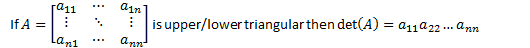(Note: Every RREF matrix is upper triangular.)Q: What happens to the determinant of a matrix if we apply EROs to it?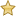Theorem: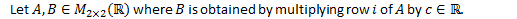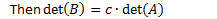Proof: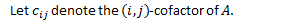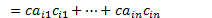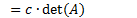Theorem: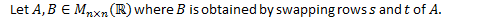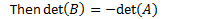Proof: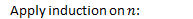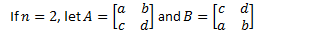(only possible row swap)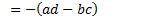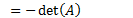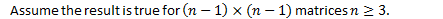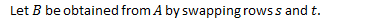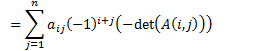(by inductive hypothesis)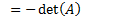Q.E.D.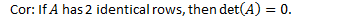Theorem: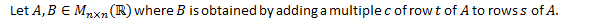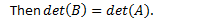Proof: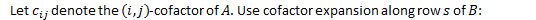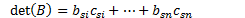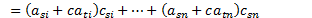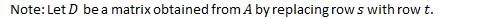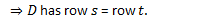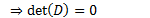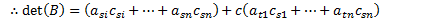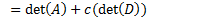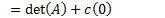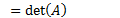Eg.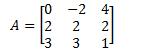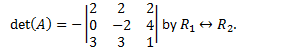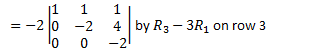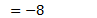Eg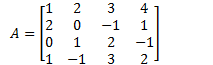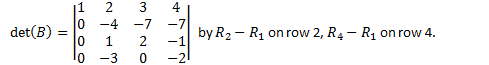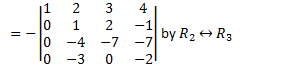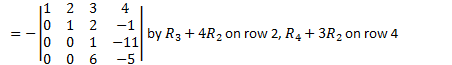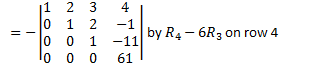Note: We can simulate an ERO by multiplying a matrix by an elementary matrix on the left.Theorem: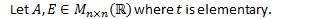Proof: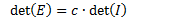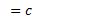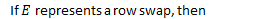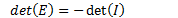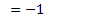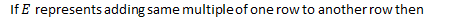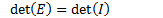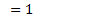Theorem (new property for the invertible Matrix Theorem):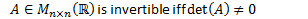Created by Tim Pei with Microsoft OneNote 2010
One place for all your notes and information